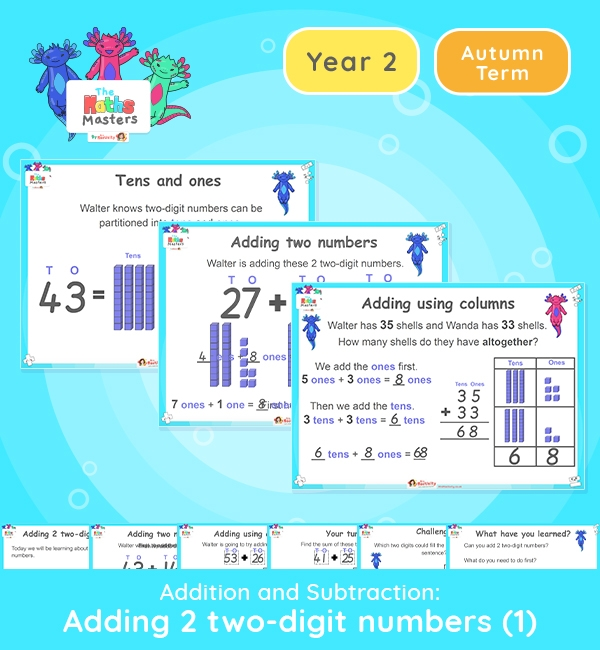# Year 2 | Adding 2 Two-Digit Numbers Lesson Presentation## Year 2 Addition and Subtraction resources

Aligned with the maths mastery approach, this Year 2 | Adding 2 Two-Digit Numbers Lesson Presentation is fully editable, and is designed for the Year 2 maths curriculum covering the following maths objectives for the autumn term:

This lesson focuses on adding 2 two-digit numbers without crossing a tens boundary.

NC Links: Recall and use addition and subtraction facts to 20 fluently and derive and use related facts to 100. Add and subtract numbers using concrete objects, pictorial representations and mentally, including: two two-digit numbers

Solve problems with addition and subtraction: using concrete objects and pictorial representations.

Year 1 Conceptual Prerequisites: Add and subtract within 10. Know that a multiple of 10 is made up of a number of tens.

2AS-4 Add and  subtract within 100 by applying related one-digit addition and subtraction facts: add and subtract any 2 two-digit numbers

TAF Statements:     Working Towards: Add and subtract (one-digit numbers) explaining their method verbally, in pictures, or using apparatus. Recall at least four of the six number bonds for 10 and reason about associated facts.

Working At: Recall all the number bonds to and within 10, and use these to reason with a calculate bonds to and within 20, recognising associated additive relationships.

Greater depth – Using reasoning about numbers and relationships to solve more complex problems and explain their thinking.

Aligned with the order of teaching of the White Rose Maths scheme of work, use this to help your class get to grips with each mathematical concept. This lesson presentation also includes varied fluency activities, problem solving, and mathematical discussion questions too.

Explore our other  year 2 addition and subtraction resources.

## Recently Viewed